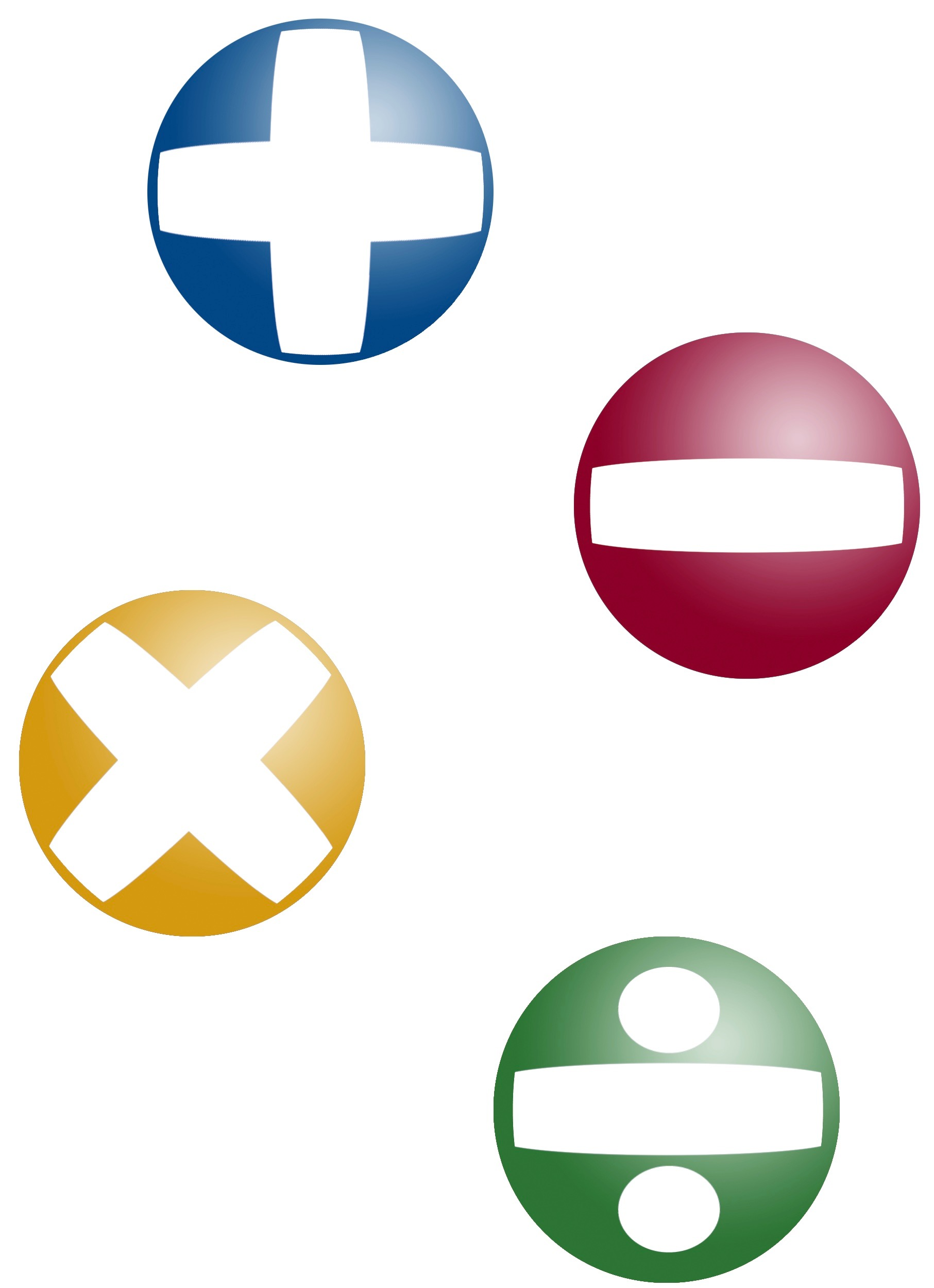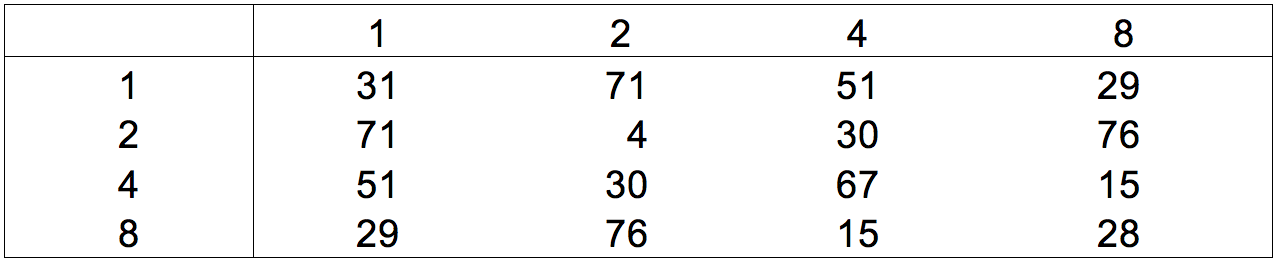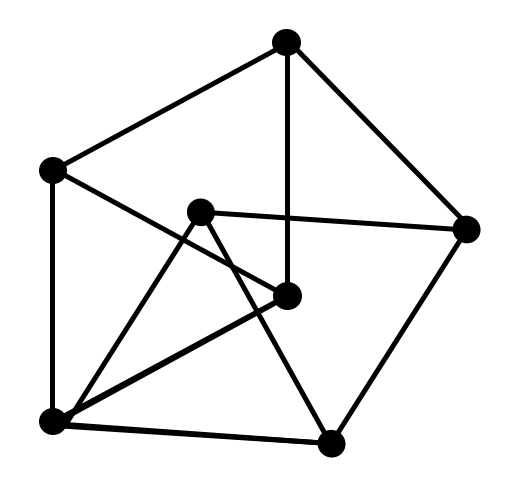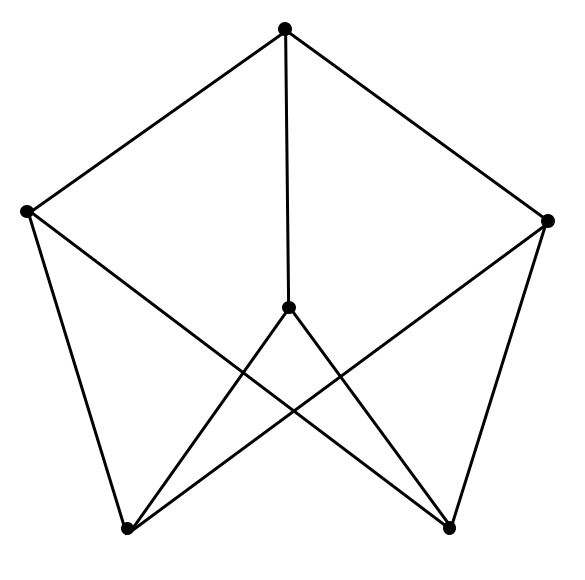## ________________

Two puzzles with numbers are on the coach’s workout for today.

## ________________### Maximization

Use the digits 1, 2, 3, and 4 once and only once to make a mathematical expression. You may use +, -, ×, ÷, exponents, decimal points, and parentheses. No roots, factorials, repeating decimals, or other mathematical functions are permitted.

What is the largest mathematical expression possible using these rules?

### Multiplication Table

Each digit in the multiplication table below represents a digit other than itself.

Create a new table with correct digits.## Solutions to week 133

In Maximizing Links, the maximum number of links is 9. For Seven Points, see the diagram below. All lengths shown are 1 foot.